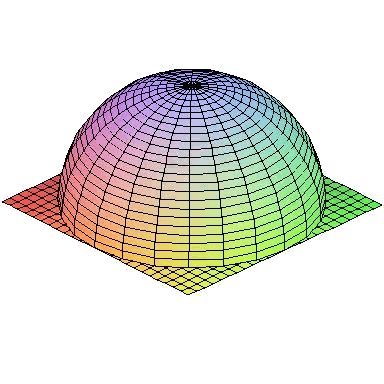# Volumes calculation using Gauss' theorem

As with what it is done with Green's theorem, we will use a powerful tool of integral calculus to calculate volumes, called the theorem of divergence or the theorem of Gauss.

This theorem says that if we have a solid $$D$$ limited by a closed surface $$S$$ and $$\vec{F}(x,y,z)$$ is a vector field, then:

$$\int_S \vec{F} \ d\vec{S} = \iiint_V \text{div}(\vec{F}) \ dx \ dy \ dz$$$where, if $$\vec{F}(x,y,z)=(P(x,y,z),Q(x,y,z),R(x,y,z))$$, then $$\text{div}(\vec{F})=P_x+Q_y+R_z$$ is the divergence of the field. Let's remember that to calculate the first term, if $$r(u, v)$$ is a parametrization of the surface, $$\int_S \vec{F} \ d\vec{S}=\iint \vec{F}\big( r(u,v) \big) \cdot (r_u\times r_v) \ du \ dv$$$

where $$r_u\times r_v$$ denotes the vector product of the derivatives of the parametrization with respect to $$u$$ and $$v$$.

Therefore, if we find a field whose divergence is equal to $$1$$, on the right term we will have the volume of the solid and, therefore, we have a method to calculate volumes.

The most typical fields that satisfy what we need are:

• $$\vec{F}(x,y,z)=(x,0,0)$$
• $$\vec{F}(x,y,z)=(0,y,0)$$
• $$\vec{F}(x,y,z)=(0,0,z)$$
• $$\vec{F}(x,y,z)=\dfrac{1}{3}(x,y,z)$$

For example, we are going to calculate the volume delimited by half a sphere and the equatorial plane, which is:From Gauss’ theorem, we can integrate one of the fields given along the surface that closes the volume up.

We will use the following parametric form, using spherical coordinates:

$$\begin{array}{l} x=\sin\theta\cos\varphi \\ y=\sin\theta\sin\varphi \\ z=\cos\theta \end{array} \qquad \begin{array}{l} \theta\in \big[0,\dfrac{\pi}{2}\big] \\ \varphi\in[0,2\pi] \end{array}$$$Let's calculate the vectors and the vector product: $$\left. \begin{array}{l} r_\theta = (\cos\theta\cos\varphi,\sin\varphi\cos\theta,-\sin\varphi) \\ r_\varphi=(-\sin\theta\sin\varphi,\sin\theta\cos\varphi,0) \end{array} \right\} \Rightarrow$$$

$$\begin{array}{rl} \Rightarrow \ r_\theta \times r_\varphi =& \begin{vmatrix} i & j & k \\ \cos\theta\cos\varphi & \sin\varphi\cos\theta & -\sin\varphi\\ -\sin\theta\sin\varphi & \sin\theta\cos\varphi & 0 \end{vmatrix} \\ =& \big( \sin^2\theta\cos\varphi, \sin^2\theta\sin\varphi, \sin\theta\cos\theta\big) \end{array}$$$Taking the vector field $$F(x,y,z)=(0,0,z)$$, we have: $$\begin{array}{rl} \int_S (0,0,z) \ d\vec{S} =& \int_0^{\frac{\pi}{2}} \int_0^{2\pi} (0,0,\cos\theta)\cdot \big( \sin^2\theta\cos\varphi, \sin^2\theta\sin\varphi, \sin\theta\cos\theta\big) \ d\varphi \ d\theta \\ =& \int_0^{\frac{\pi}{2}} \int_0^{2\pi} \cos^2\theta \cdot \sin\theta \ d\varphi \ d\theta = \int_0^{\frac{\pi}{2}} \cos^2\theta \cdot \sin\theta \Big( \int_0^{2\pi} d\varphi \Big) \ d\theta \\ =& 2\pi \int_0^{\frac{\pi}{2}} \cos^2\theta \cdot \sin\theta \ d\theta = 2\pi \Big[ \dfrac{-\cos^3\theta}{3} \Big]_0^{\frac{\pi}{2}}= \dfrac{2}{3}\pi \end{array}$$$

Now we must integrate the same field on the low lid since the theorem is valid for closed surfaces. A parametrization of the circle is:

$$\begin{array}{l} x=r\cos(t) \\ y=r\sin(t) \\ z=0 \end{array} \qquad \begin{array}{l} t\in[0,2\pi] \\ r\in[0,1] \end{array}$$$Let's calculate also the vectors and its vector product: $$\left. \begin{array}{l} r_r = (-r\sin(t),r\cos(t),0) \\ r_t=(\cos(t),\sin(t),0) \end{array} \right\} \Rightarrow$$$

$$\Rightarrow \ r_t \times r_r = \begin{vmatrix} i & j & k \\ -r\sin(t) & r\cos(t) & 0 \\ \cos(t) & \sin(t) & 0 \end{vmatrix} = ( 0, 0, -r^2)$$$Now, we have: $$\int_C (0,0,z) \ d\vec{S} = \int_0^{2\pi} \int_0^1 (0,0,0)\cdot (0,0,-r^2) \ dr \ dt =0$$$

Therefore,

$$\begin{array}{rl} \text{Vol}(E)=&\iiint_S 1 \ dx\ dy\ dz= \iiint_E \text{div}(0,0,z) \ dx\ dy\ dz \\ =& \int_S (0,0,z) \ d\vec{S}+ \int_C (0,0,z)\ d\vec{S} = \dfrac{2}{3}\pi +0= \dfrac{2}{3}\pi \end{array}$$\$